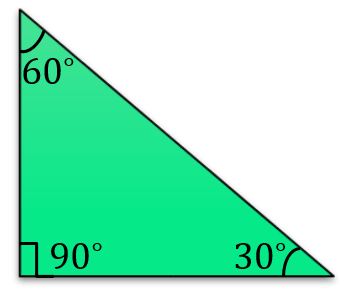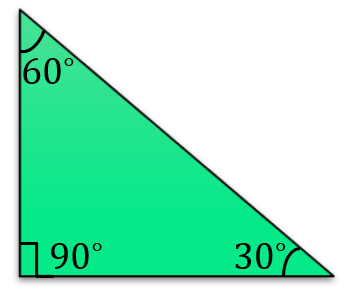1
visibility

What kind of triangle is this?• Acute Triangle

• Obtuse Triangle

• Right Triangle

The correct answer is "Right Triangle".

Solution:

Consider the triangle below.Notice that one of the three angles of this triangle is exactly 90°.

So, it is a Right Triangle.